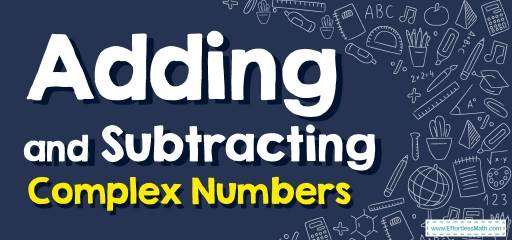# How to Add and Subtract Complex Numbers? (+FREE Worksheet!)## Step by step guide to add and subtract the complex numbers

• A complex number is expressed in the form $$a+bi$$, where $$a$$ and $$b$$ are real numbers, and $$i$$, which is called an imaginary number, is $$a$$ solution of the equation $$x^2=-1$$
• For adding complex numbers: $$(a+bi)+(c+di)=(a+c)+(b+d)i$$
• For subtracting complex numbers: $$(a+bi)-(c+di)=(a-c)+(b-d)i$$

### Adding and Subtracting Complex Numbers – Example 1:

Solve: $$10+(-5-3i)-2$$

Solution:

Remove parentheses: $$10+(-5-3i)-2=10-5-3i-2$$
Combine like terms: $$10-5-2=10-7=3$$

Then: $$10-5-3i-2=3-3i$$

The Absolute Best Book to Ace Pre-Algebra

### Adding and Subtracting Complex Numbers – Example 2:

Solve: $$-3+(4i)+(9-2i)$$

Solution:

Remove parentheses: $$-3+(4i)+(9-2i)=-3+4i+9-2i$$
Combine like terms: $$-3+9=6 , 4i- 2i=2i$$

Then: $$-3+4i+9-2i=6+2i$$

### Adding and Subtracting Complex Numbers – Example 3:

Solve: $$(-8+2i)+(-8+6i)$$

Solution:

Remove parentheses: $$(-8+2i)+(-8+6i)=-8+2i-8+6i$$
Combine like terms: $$-8-8=-16 , 2i+6i=8i$$
Then: $$-8+2i-8+6i=-16+8i$$

The Absolute Best Book to Ace the College Algebra Course

### Adding and Subtracting Complex Numbers – Example 4:

Solve: $$(6-3i)-(-8-7i)$$

Solution:

Remove parentheses by multiplying the negative sign to the second parenthesis: $$(6-3i)-(-8-7i)=6-3i+8+7i$$
Combine like terms: $$6+8=14 , -3i+7i=4i$$

Then: $$6-3i+8+7i= 14+ 4i$$

## Exercises for Adding and Subtracting Complex Numbers

### Simplify.

• $$\color{blue}{– 8 + (2i) + (– 8 + 6i)}$$
• $$\color{blue}{12 – (5i) + (4 – 14i)}$$
• $$\color{blue}{– 2 + (– 8 – 7i) – 9}$$
• $$\color{blue}{(– 18 – 3i) + (11 + 5i)}$$
• $$\color{blue}{(3 + 5i) – (8 + 3i)}$$
• $$\color{blue}{(8 – 3i) – (4 + i)}$$

• $$\color{blue}{– 16 + 8i}$$
• $$\color{blue}{16 – 19i}$$
• $$\color{blue}{–19 – 7i}$$
• $$\color{blue}{–7 + 2i}$$
• $$\color{blue}{-5 + 2i}$$
• $$\color{blue}{4 – 4i}$$

The Best Books to Ace Algebra

### What people say about "How to Add and Subtract Complex Numbers? (+FREE Worksheet!) - Effortless Math: We Help Students Learn to LOVE Mathematics"?

No one replied yet.

X
45% OFF

Limited time only!

Save Over 45%

SAVE $40 It was$89.99 now it is \$49.99